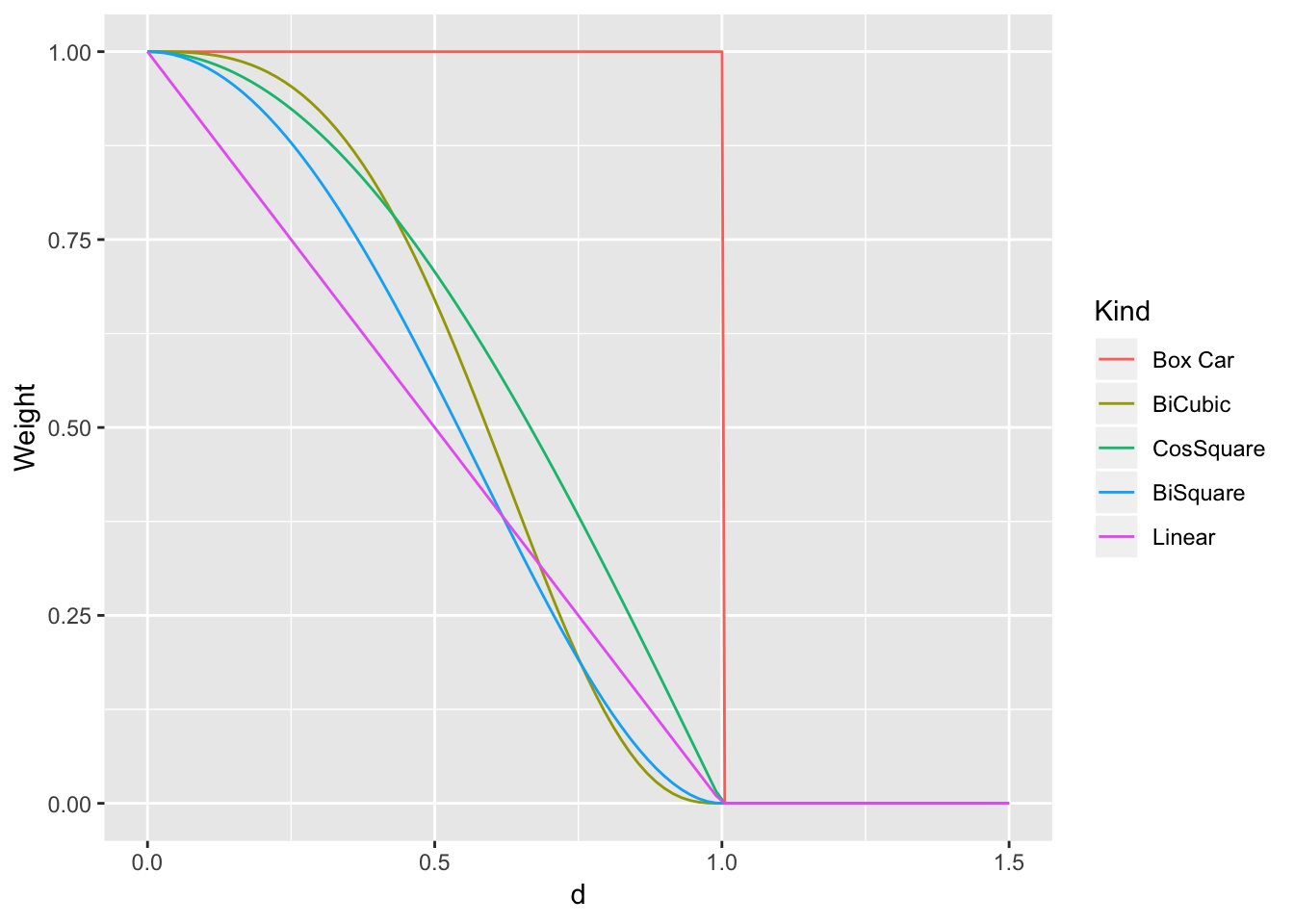## Introduction

The aim here is to provide an approach to geographically weighted approaches that fits neatly into the ‘tidy data’ framework provided by the dplyr package and other related ones. Initially, this requires the tidyverse group of packages to be loaded from the library:

library(tidyverse)

The way this is achieved is to store the geographical weighting as a sparse matrix - but essentially in data frame (or tibble) format. Suppose we have a source data set $$S$$ (with each record having a geographical location) and a target data set $$T$$ (also with each record having a geographical location. Sometimes the source and target data sets are the same. Then there will be a distance between each element in $$S$$ and each element in $$T$$, giving $$n(S) \times n(D)$$ distances. These can be stored in a three column data frame of the form:

Source data object ID Target data object ID Distance
SID001 TID001 1.5km
SID001 TID002 6.4km
$$\vdots$$ $$\vdots$$ $$\vdots$$

If $$S$$ and $$T$$ are both large data sets, then this may be a problem. However, here, the weights (which depend on the distances) are of more importance. The weights are a function of distance, as well as some paramter to control the window width. If we use distance-based weights to begin with, the parameter will be called $$h$$ and will represent the radius within which the weights are non-zero.

Some examples are

Name Formula when $$d<h$$ Formula when $$d \ge h$$ Slope when $$d \nearrow h$$
Box Car $$1$$ $$0$$ $$-\infty$$
Linear $$1 - \frac{d}{h}$$ $$0$$ $$-\frac{1}{h}$$
BiSquare $$\left(1 - (\frac{d}{h})^2\right) ^2$$ $$0$$ $$0$$
BiCubic $$\left(1 - (\frac{d}{h})^3\right) ^3$$ $$0$$ $$0$$
CosSquare $$\cos^2 \left( \frac{\pi d}{2h} \right)$$ $$0$$ $$0$$

and their graphs are as below when $$h = 1$$As seen in the table, not all of these are smooth when $$d=h$$ - in particular the Box Car and Linear weighting functions are not. However, these are all weighting schemes for which the weight is zero once the the distance between geographical objects exceeds $$d$$. This is where a sparse storage method is useful. These weights will be used for weighted sums, means and so on, and anything with a zero weight is excluded. Thus, to save space, the tabular form suggested above, but with weights rather than distances - and more specifically only non-zero weights will be used. Thus the table will be:

Source data object ID Target data object ID Weight
SID001 TID001 0.41
SID001 TID002 0.03
$$\vdots$$ $$\vdots$$ $$\vdots$$

with only rows for which the weight is non zero included. Omitted source/target ID combinations are assumed to have a weight of zero.

Here is some R code to create functions for these weighting schemes:

bisquare <- function(x,h) ifelse(x<h,(1 - (x/h)^2)^2,0)

bicube <- function(x,h) ifelse(x<h,(1 - (x/h)^3)^3,0)

boxcar <- function(x,h) ifelse(x<h,1,0)

linear <- function(x,h) ifelse(x<h,1-x/h,0)

cossquare <- function(x,h) ifelse(x < h, cos(x*pi/(h*2))^2, 0)

Next, here is a helper function to create a sparse weight table, given two ‘simplefeatures’ (sf) objects. You will need to specify the ID variables and the name of each of the objects, and the winsow size $$h$$. Don’t worry too much about how it works for now. The function wt_tbl will be treated as a method (here for example it is just defined for sf objects, but later a matrix method will be defined).

wt_tbl <- function(x,...) UseMethod("wt_tbl")

wt_tbl.sf <- function(sfx,sfy,idx,idy,
wt=wt,dmax=Inf,wfun = bisquare, h, ...) {
idx <- enquo(idx)
idy <- enquo(idy)
wt =enquo(wt)
dmat <- st_distance(sfx,sfy,...) %>% units::drop_units()
colnames(dmat) <- sfy %>% select(!!idy) %>%
st_set_geometry(NULL) %>% .[]
rownames(dmat) <- sfx %>% select(!!idx) %>%
st_set_geometry(NULL) %>% .[]
res <- dmat %>% as_tibble(rownames=quo_name(idx)) %>%
gather(key=!!idy,value= !! wt, - !! idx) %>% filter(!! wt <= dmax)
if (missing(h)) {
kfun <- partial(wfun,h=dmax) }
else {
kfun <- partial(wfun,h=h)
}
res %>% mutate(!! wt := kfun(!! wt))
}

Next, it can be demonstrated. Read in some data relating to house prices and convert it to an sf object.

library(sf)

rename(house_ID=ID) %>%
mutate(price = price/1000, house_ID=sprintf('H%04d',house_ID)) %>%
st_as_sf(coords=c('east','north'),crs=27700)
head(hp)
Simple feature collection with 6 features and 3 fields
geometry type:  POINT
dimension:      XY
bbox:           xmin: 514600 ymin: 169200 xmax: 547900 ymax: 189600
epsg (SRID):    27700
proj4string:    +proj=tmerc +lat_0=49 +lon_0=-2 +k=0.9996012717 +x_0=400000 +y_0=-100000 +ellps=airy +towgs84=446.448,-125.157,542.06,0.15,0.247,0.842,-20.489 +units=m +no_defs
# A tibble: 6 x 4
house_ID price fl_area        geometry
<chr>    <dbl>   <int>     <POINT [m]>
1 H0001    107        50 (523800 179700)
2 H0002     55.5      66 (533200 170900)
3 H0003    103        90 (514600 175800)
4 H0004    187       125 (516000 171000)
5 H0005     43        50 (533700 169200)
6 H0006     70.0      95 (547900 189600)

A straighforward map is now possible:

library(ggplot2)
library(ggspatial)
ggplot(hp,aes(col=price)) +
annotation_map_tile(type='osm',zoomin=-1) + geom_sf() +
scale_color_viridis_c(trans='log10')